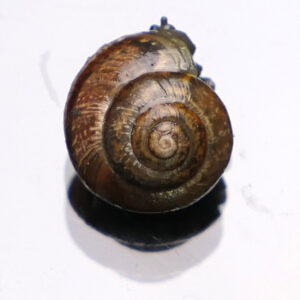# Silver jewelry with Fibonacci spiral & Golden spiral.

Fibonacci spiral & Golden spiral as a silver jewelry is now available in my web shop.Fibonacci numbers are numbers where each number is the sum of the two previous Fibonacci numbers; 0, 1, 1, 2, 3, 5, 8, 13, 21, 34…

A Fibonacci spiral starts with a rectangle partitioned into 2 squares. In each step, a square the length of the rectangle’s longest side is added to the rectangle.

The Fibonacci sequence is related to the golden ratio. The golden ratio  φ is a mathematical constant ≈1.618. A rectangle whose sides relate to the golden ratio is called the golden rectangle. A golden rectangle can be divided into a square and a smaller golden rectangle. By repeatedly dividing the smaller rectangle in the same way, you get a figure in which the so-called golden spiral, can be drawn in.

The Fibonacci number occurs in spiral structures in nature.The number of spirals calculated counterclockwise or clockwise, respectively, in such structures constitute two successive Fibonacci numbers.